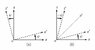# Is the rotation angle in Minkowski's diagram real or imaginary?

• gene1721

#### gene1721

In Figure_1(b) I have depicted a simplified version of Minkowski's diagram, where

$$\beta = \frac{v}{c}= \tanh \psi= - i \tan i \psi= \frac{( e^{\psi} - e^{-\psi})}{( e^{\psi} + e^{-\psi})}$$,

the rotation of $$(x',t')$$-axes being defined as imaginary (considering x and t real, and c=1).

However, I have found books where this rotation is considered as real, while the rotation in Figure_1(a) is shown as imaginary (considering x real, t imaginary, and c=1).

Could you explain which rotation is real and which imaginary?

Figure_1 is here!

#### Attachments

•Figure_1.jpg
12.8 KB · Views: 822
Doing a Lorentz transformation (boost) by a velocity v to some 4-vector is equivalent to rotate it an imaginary angle $$\chi$$ such that:

$$\chi = arctan( v )$$

Thanks Kuon!

If I understand correctly, you come with a third possibility, right?Could you explain which rotation is real and which imaginary?
That depends on how you define "rotation". I wouldn't call a Lorentz boost a "rotation". I don't mind calling it a "hyperbolic rotation" or a "Minkowski orthogonal transformation" but calling it a "rotation" is a bit weird in my opinion.

There's no need to bring imaginary numbers into this.

The angle of a rotation is always real and can be defined by writing the matrix as

$$\begin{pmatrix}\cos\theta & -\sin\theta\\ \sin\theta & \cos\theta\end{pmatrix}$$

A 2×2 matrix represents a proper rotation if and only if it's orthogonal and has determinant 1. Alll 2×2 matrices with those properties can be put in the form above, with different values of $\theta$.

The rapidity of a Lorentz boost in 1+1 dimensions is always real and can be defined by writing the matrix as

$$\begin{pmatrix}\cosh\theta & -\sinh\theta\\ -\sinh\theta & \cosh\theta\end{pmatrix}$$

A 2×2 matrix $\Lambda$ represents a proper orthochronous Lorentz boost if and only if it satisfies $\Lambda^T\eta\Lambda=\eta$, $\det\Lambda=1$, and $\Lambda_{00}\geq 1$. (That's just its upper left component). All 2×2 matrices with those properties can be put in the form above, with different values of $\theta$. This $\theta$ is usually referred to as the "rapidity" of the Lorentz transformation, not the "angle", but I wouldn't mind calling it the "hyperbolic angle" or even the "angle of a hyperbolic rotation". I just don't want to call it a "rotation angle", as you did in the thread title.

Note that you can't turn one of these matrices into the other just by substituting $\theta\rightarrow i\theta$. That's why it doesn't quite make sense to describe a Lorentz transformation as a rotation by an imaginary angle.

Last edited:
That's why it doesn't quite make sense to describe a Lorentz transformation as a rotation by an imaginary angle.

Scott Walter (http://www.univ-nancy2.fr/DepPhilo/walter/), who published several papers on Minkowski and his diagram, wrote on page 9 of this article http://www.univ-nancy2.fr/DepPhilo/walter/papers/nes.pdf

... Minkowski retained the geometric interpretation of Lorentz transformation ... In doing so, he elaborated the notion of velocity as a rotation in four-dimensional space. He introduced a formula for the frame velocity $$q$$ in terms of the tangent of an imaginary angle $$i \psi$$, such that

$$q = - i \tan i \psi= \frac{( e^{\psi} - e^{-\psi})}{( e^{\psi} + e^{-\psi})}$$.

Minkowski could very well have expressed frame velocity in the equivalent form $$q = \tanh \psi$$, where the angle of rotation is real instead of imaginary, and all four space-time coordinates are real. He did not do so, but used the imaginary rotation angle $$i \psi$$ to express the special Lorentz transformation in the trigonometric form:

$$x_1'= x_1,~~x_2'= x_2,~~x_3'= x_3 \cos i \psi + x_4 \sin i \psi,~~x_4'= - x_3 \sin i \psi+ x_4 \cos i \psi$$.

The use of circular functions here underscores the fact that a special Lorentz transformation is equivalent to a rotation in the $$( x_3 x_4 )$$--plane. Likewise, by expressing velocity in terms of an imaginary rotation, Minkowski may have...

Minkowski's preference for circular functions may be understood in relation to his project to express the laws of physics in four-dimensional terms. ... Expressing the Lorentz transformation as a hyperbolic rotation would have obscured the connection for physicists.

We can see now not only that it does make sense to describe a Lorentz transformation as a rotation by an imaginary angle, but to also inquire why would Minkowski really want to use the imaginary rotation. (I have to say that I buy only partially into Walter's explanation!)

Trying to understand why wanted Minkowski to use the imaginary angle, I found in different sources three ways (including Koun's version!) to explain the rotation angle in Minkowski's diagram:

1. x real, t real and the rotation angle real;
2. x real, t real and the rotation angle imaginary;
3. x real, t imaginary and the rotation angle imaginary.

So, given that Minkowski didn't want to use version 1, which between version 2 and version 3 is the correct one?

Last edited by a moderator: